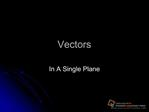DownloadDownload PresentationVectors

# Vectors

Download Presentation## Vectors

- - - - - - - - - - - - - - - - - - - - - - - - - - - E N D - - - - - - - - - - - - - - - - - - - - - - - - - - -
##### Presentation Transcript

Slide 1:Vectors

In A Single Plane

Slide 2:Vector Representation

Have you ever drawn a treasure map as a child? Drawn a map to you home for someone else? Vector quantities are represented by arrows that point in the direction of the quantity

Slide 3:Arrows for Vectors

The length of the arrow � magnitude of quantity ( drawn to scale) The direction of the arrow � direction of quantity (reference point) � you need a coordinate system or frame of reference (diagram)

Slide 4:Three ways to indicate direction of vectors Angles 0-360 degrees NSEW [N 30� E] Bearings

Can�t add apples and oranges You can only add vectors that represent the same quantity and are drawn with the same scale (displacements, forces) Resultant � sum of all vectors

Set up coordinate system Place vector A Place the tail of vector B at the tip of vector A (tip to tail) Repeat step 3 if more than one vector Draw a vector from the tail of the first vector to the tip of the last vector. Label this as you resultant Use a ruler to measure the length of the resultant Use a protractor to measure the angle between the resultant and the horizontal axis Do practice problems page 93

Slide 7:Subtracting Vectors

Subtracting Vectors graphically ?d = d2 � d1 ?v = v2 � v1 A-B same as A + (-B) Negative vectors has the same magnitude and opposite direction

Slide 8:Steps for Subtracting Vectors

Set up coordinate system Place vector A Place the tail of negative vector B at the tip of vector A (tip to tail) Draw a vector from the tail of the first vector to the tip of the last vector. Label this as you resultant Use a ruler to measure the length of the resultant (magnitude) Use a protractor to measure the angle between the resultant and the horizontal axis Do practice problems page 98

Slide 9:Multiplying and Dividing Vectors

What happens to a vector when it is multiplied or divided by a scalar value? V = ?d / t When displacement (vector) is divided by time (scalar) the resulting vector has a new magnitude and unit but the direction remain the same.

Slide 10:Relative Velocity

Analyze quantitatively, the motion of an object is relative to different reference points Eg: stopped at a red light, some times it feels like you�re moving backwards�are you? Vector addition is a critical tool in calculating relative velocities

Slide 11:Pilot � ground and air as frames of reference You must account for both the motion of the plane relative to the air and the air relative to the ground Apply velocity vectors for each Plane to air velocity + air to ground velocity plane velocity to ground